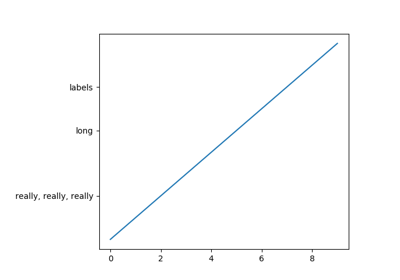# matplotlib.figure.SubplotParams¶

class `matplotlib.figure.``SubplotParams`(left=None, bottom=None, right=None, top=None, wspace=None, hspace=None)[source]

Bases: `object`

A class to hold the parameters for a subplot.

All dimensions are fractions of the figure width or height. Defaults are given by `rcParams["figure.subplot.[name]"]`.

Parameters: left : float The left side of the subplots of the figure. right : float The right side of the subplots of the figure. bottom : float The bottom of the subplots of the figure. top : float The top of the subplots of the figure. wspace : float The amount of width reserved for space between subplots, expressed as a fraction of the average axis width. hspace : float The amount of height reserved for space between subplots, expressed as a fraction of the average axis height.
`update`(left=None, bottom=None, right=None, top=None, wspace=None, hspace=None)[source]

Update the dimensions of the passed parameters. None means unchanged.

## Examples using `matplotlib.figure.SubplotParams`¶